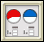Homework HelpMultiple-Choice Skills Practice Active Math Online Did You Know?Vocabulary QuizVideo TutorBits And Pieces II   Homework Help Bits And Pieces II Multiple-Choice Skills Practice Bits And Pieces IIVocabulary QuizBits And Pieces III  Homework Help Bits And Pieces III Multiple-Choice Skills Practice Bits And Pieces IIIVocabulary Quiz

Bits and Pieces I, II, III

Decimals

Fractions

• Jamit Fractions Lessons & Games
• AAAMath Fractions, Ratios
• Illuminations Fractions
• FunSchool Action Fraction
• BBC Bitesize Fractions, Ratios
• Cool Math:
• National Library of Virtual Manipulatives
•Fraction Pieces – Work with parts and wholes to learn about fractions.Fractions - Adding – Illustrates what it means to find a common denominator and combine.Fractions - Comparing – Judge the size of fractions and plot them on a number line.Fractions - Equivalent – Illustrates relationships between equivalent fractions.Fractions - Rectangle Multiplication – Visualize and practice multiplying fractions using an area representation.
• Fraction Cafe

Percents

• Worsley School Percents
• AAAMath Percents
• BBC Bitesize Percents
• National Library ofVirtual Manipulatives
•Percent Grids – Represent, name, and explore percentages using hundreds grids.Percentages – Discover relationships between fractions, percents, and decimals.Bits and Pieces I

(University of Michigan Overview)
Concept with Explanation
Selected Homework from ACE
In Bits and Pieces I students will investigate and make sense of rational numbers and operations with rational numbers. The investigations in this unit will help students to:

• Build an understanding of fractions, decimals, and percents and the relationships between and among these concepts and their representations;
• Develop ways to model situations involving fractions, decimals, and percents;
• Understand and use equivalent fractions to reason about situations;
• Compare and order fractions;
• Move flexibly between fraction, decimal, and percent representations;
• Use benchmarks such as 0, 1/2 , 1, and 1/4 to help estimate the size of a number or sum;
• Develop and use benchmarks that relate different forms of representations of rational numbers (for example, 50% is the same as 1/2 and 0.5);
• Use physical models and drawings to help reason about a situation;
• Look for patterns and describe how to continue the pattern;
• Use context to help reason about a situation; and
• Use estimation to understand a situation..

Bits and Pieces II
Concept with Explanation
Selected Homework from ACE
Bits and Pieces II was created to help students make sense of one aspect of rational number: fraction operations. The investigations in this unit will help students to:

• Use benchmarks and other strategies to estimate the reasonableness of results of operations with fractions;
• Develop ways to model sums, differences, products, and quotients with areas, strips, and number lines;
• Use estimates and exact solutions to make decisions;
• Look for and generalize patterns in numbers;
• Use knowledge of fractions and equivalence of fractions to develop algorithms for adding, subtracting, multiplying and dividing fractions;
• Recognize when addition, subtraction, multiplication, and division is the appropriate operation to solve a problem;
• Write fact families to show the inverse relationship between addition and subtraction, and between multiplication and division;
• Solve problems using arithmetic operations on fractions; and
• Look for and generalize patterns.

Bits and Pieces III
Concept with Explanation
Selected Homework from ACE
Bits and Pieces III, along with Bits and Pieces I and Bits and Pieces II, completes the development of meaning of, and operations on, fractions, decimals, and percents. This unit will help students to:

• Connect to what students already know about operations on fractions and whole numbers;
• Develop and use benchmarks and other strategies to estimate the answers to computations with decimals;
• Develop meaning of and algorithms for operations with decimals;
• Use the relationship between decimals and fractions to develop and understand why decimal algorithms work;
• Use the place value interpretation of decimals to make sense of short-cut algorithms for operations;
• Generalize number patterns to help make sense of decimal operations;
• Understand when addition, subtraction, multiplication or division is an appropriate operation to use to solve a problem;
• Understand that decimals are often associated with measurements in real world situations;
• Solve problems using operations on decimals;
• Use understanding of operations and the meaning of percents to solve percent problems of the form a% of b equals c for any one of the variables a, b, or c; and
• Create and interpret circle graphs